# High School Chemistry : Help with Radioactive Decay

## Example Questions

← Previous 1

### Example Question #1 : Nuclear Chemistry

What is the cause for radioactive decay?

Instability in the electron cloud

Lack of energy in an atom

Ionization of an atom

Instability in the nucleus

All of these can cause radioactive decay

Instability in the nucleus

Explanation:

Radioactive decay is the result of an unstable nucleus in an atom. When an atom contains more neutrons than the nucleus can handle it will undergo radioactive decay, leading to emission of different particles such as alpha particles or beta electrons. This can occur in ground state elemental atoms, but is most commonly seen in radioactive isotopes. Isotopes contain the same number of protons as any other atom of a given element, but often contain a greater number of neutrons, leading to instability. These neutrons will be lost via radioactive decay in order for the atom to reach the ground state, the most stable isotope.

Ionization results in a charged particle via electron transfer, but does not contribute to radioactivity.

### Example Question #1 : Help With Radioactive Decay

Which type of radioactive decay does not emit any physical matter?

Electron capture

Alpha decay

Gamma decay

Beta decay

Positron decay

Gamma decay

Explanation:

Gamma radiation is the only form of radioactive decay that does not emit a physical particle from the atomic nucleus. It is simply the release of energy in the form of high energy gamma rays, which do not carry any mass. These rays are more powerful than any other electromagnetic wave known to man.

Alpha decay results in the release of a helium nucleus. Beta decay results in the release of an electron or a positron. Electron capture results in the emission of a neutron.

### Example Question #11 : Nuclear Chemistry

Fluorine-18 is a radioactive isotope of fluorine that is used in medical imaging scans. It has a half-life of approximately 110 minutes.

If a patient has a medical imaging scan using an injection of fluorine-18 at 9am, at what time will there be less than 25% of the radioactive isotope in her body?

10am

1pm

11am

9am

12pm

1pm

Explanation:

A half life is the amount of time it takes a radioactive sample to decay by 50%. To reach 25% of the initial dose would take two half lives.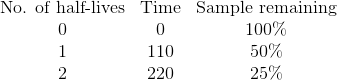It will take 220 minutes, or 3 hours and 40 minutes, for there to be 25% of the sample remaining. Since the sample was administered at 9am, this means that there will be less than 25% remaining at 1pm.

### Example Question #1 : Help With Radioactive Decay

Which of the following is not a type of nuclear particle involved in radioactive decay?

Electron

Alpha particle

Nucleolus

Beta particle

Positron

Nucleolus

Explanation:

Alpha decay, beta decay, and positron emission are all commonly recognized forms of radioactive decay. Electron capture is another form of nuclear alteration via radioactivity. The nucleolus is a biological structure found in cells, and has no relation to the atomic nuclei described in chemistry.

### Example Question #143 : Elements And Atoms

What kind of radiation has no charge or mass?

gamma

alpha

beta

delta

gamma

Explanation:

This is the definition of gamma radiation.

### Example Question #1 : Nuclear Decay

Consider the following isotope of thorium: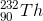What is the identity of the product following three alpha decay reactions?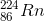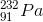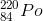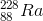Explanation:

During alpha decay, an element emits a helium nucleus with 2 neutrons and 2 protons. Thus, the atomic mass of the new element is decreased by four, and the atomic number is decreased by two.

Three subsequent alpha decays result in a new element with an atomic mass of 232 - 3(4) = 220, and a new atomic number of 90 - 3(2) = 84.

Using the periodic table, we find the element with this atomic number is polonium (Po).

### Example Question #1 : Radioactive Decay And Nuclear Chemistry

Consider the following isotope: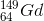What is the identity of the product after the following series of decay reactions?

alpha decay, alpha decay, electron emission, positron emission, positron emission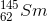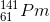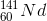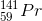Explanation:

In alpha decay, a helium nucleus is emitted, and thus the isotope loses 2 protons and 2 neutrons.

In electron emission, a neutron in the nucleus is converted into a proton and an emitted electron.

In positron emission, a proton in the nucleus is converted into a neutron and an emitted positron.

The given isotope will lose 4 protons and 4 neutrons via alpha emission, gain 1 proton and lose 1 neutron via electron emission, and lose 2 protons and gain 2 neutrons via positron emission. The result is a loss of 5 protons and 8 mass units.

Accounting for the changes in atomic mass and number, we find that the final element is 141-praseodymium.

### Example Question #2 : Help With Radioactive Decay

The half-life of a certain isotope is 20min. How long will it take for 1g of the isotope to decay to 0.25g?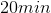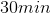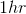None of the answer choices are correct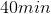Explanation:

By definition, half-life is the amount of time it takes for a radioactive substance to decrease by one-half its original value. In this question, the isotope's half life is 20min, meaning after 20min, 1g of the isotope will be reduced to 0.5g. After another 20min, 0.5g of the isotope will be reduced to 0.25g. Adding up the two 20min time frames it took to go from 1g of substance to 0.25g gives 40min.

### Example Question #1 : Help With Radioactive Decay

A radioactive isotope has a half-life ofdays. If a chemist has a sample of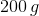, how long will it take until there isof the sample?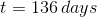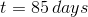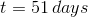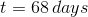Explanation:

There is an equation to calculate half-life problems, but if the sample is cut into halves every half-life, there is a simple way to find the time passed. Let's start with the total mass of the sample at time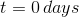. For every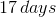the sample size will be cut in half: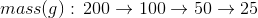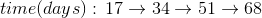After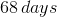there will be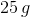of the isotope left.

### Example Question #581 : High School Chemistry

Technetium-99 is used in hospitals and has a very short half-life of about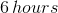. If a lab receives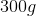of Technetium-99, how much of the sample will remain after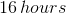?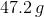None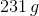Explanation:

For problems with half-life, we will use the equation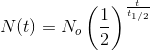Where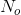is the initial mass of the sample,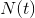is the amount left after timepasses and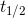is the half-life of the isotope. We have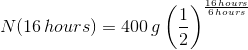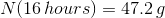← Previous 1

### All High School Chemistry Resources